# Question 2 Determine if f(x) = 4x3 – 2x is even, odd, or neither? Insufficient Information...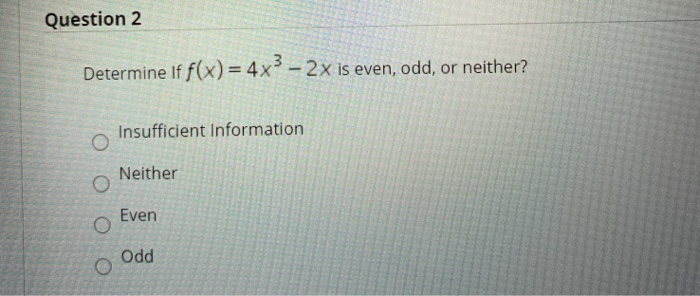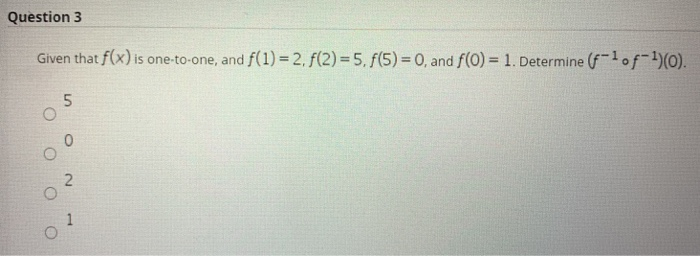Question 2 Determine if f(x) = 4x3 – 2x is even, odd, or neither? Insufficient Information O Neither Even Odd
Question 3 Given that f(x) is one-to-one, and f(1) = 2. f(2)=5. f(5) = 0, and f(0) = 1. Determine (-10f-1)0). 5 2. O 1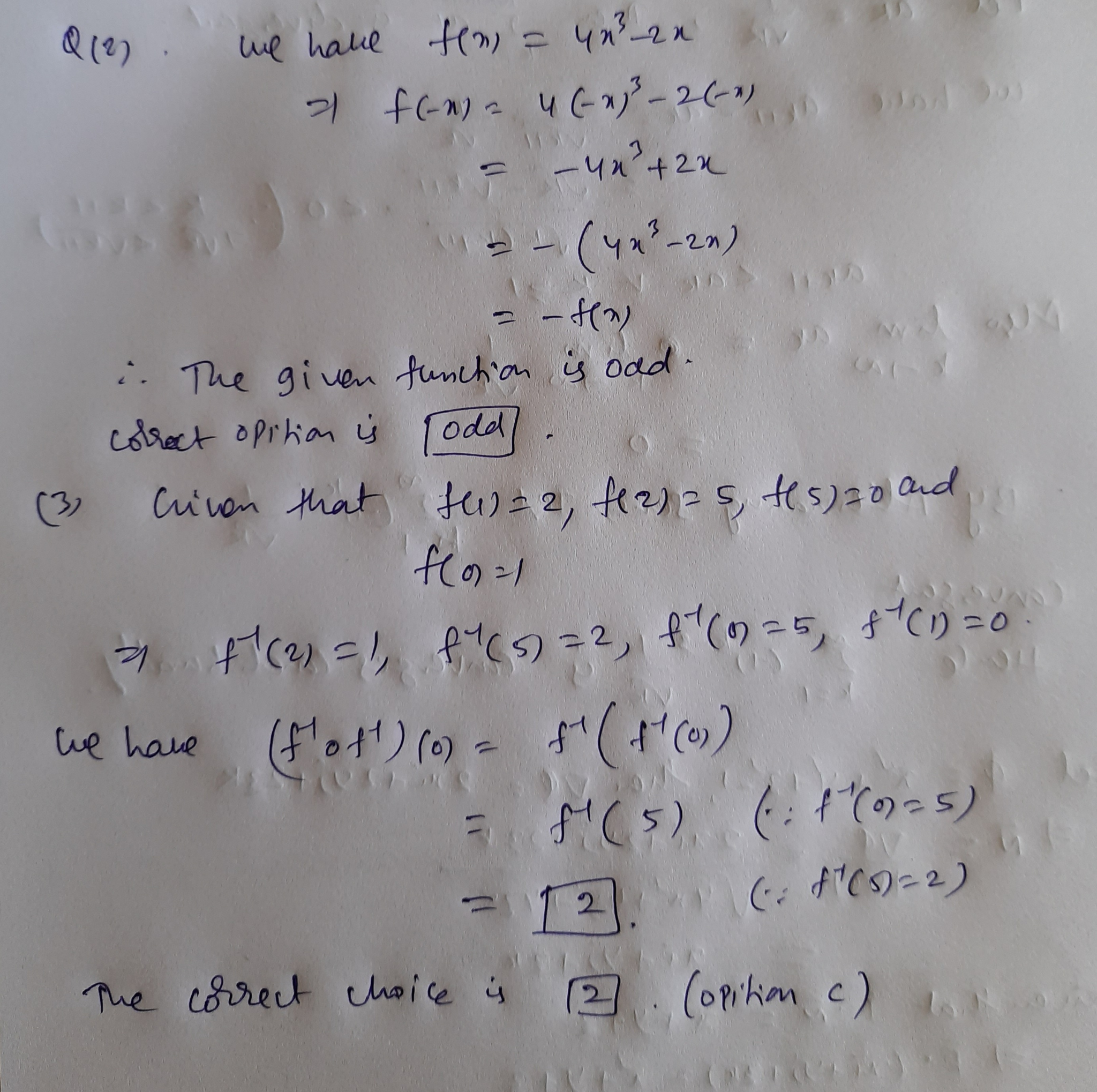##### Add Answer of: Question 2 Determine if f(x) = 4x3 – 2x is even, odd, or neither? Insufficient Information...
Similar Homework Help Questions
• ### Question 1 The graph of x4 - y = 1 is not symmetric with respect to...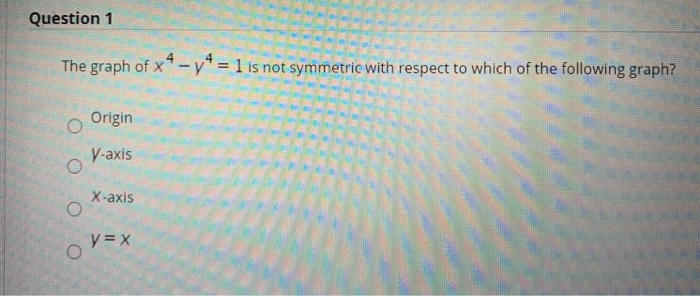Question 1 The graph of x4 - y = 1 is not symmetric with respect to which of the following graph? o Origin V-axis X-axis O y = x O Question 2 Determine if f(x)= 4x3 - 2x is even, odd, or neither? Insufficient Information Neither Even Odd Question 3 Given that f(x) is one-to-one, and f(1) = 2. f(2)=5. f(5)= 0, and f(0) = 1. Determine (f-lof-1)(0). 5 O 0 2 1

• ### Show that the function f (x) 8r 4x3 3 is even, odd, or neither f(-x) = 2 Edit f(a) is even. Click...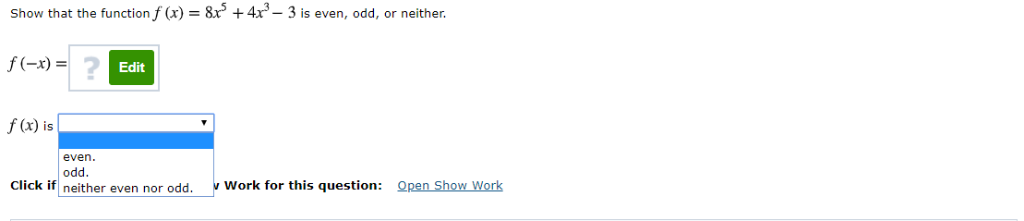Show that the function f (x) 8r 4x3 3 is even, odd, or neither f(-x) = 2 Edit f(a) is even. Click iw mether even nor od work for this question Qnan Shon Warks Work for this question: Qpen Show Work Show that the function f (x) 8r 4x3 3 is even, odd, or neither f(-x) = 2 Edit f(a) is even. Click iw mether even nor od work for this question Qnan Shon Warks Work for this question: Qpen...

• ### Let f(x) = x^4 + 2x^2 + 1 / x + 1 Find f(-x)and determine if f is even, odd, or neither

Let f(x) = x^4 + 2x^2 + 1 / x + 1Find f(-x)and determineif f is even, odd, or neither.

• ### & Detumine is if the or function f(x) = 2x² - x neither even, odd 7....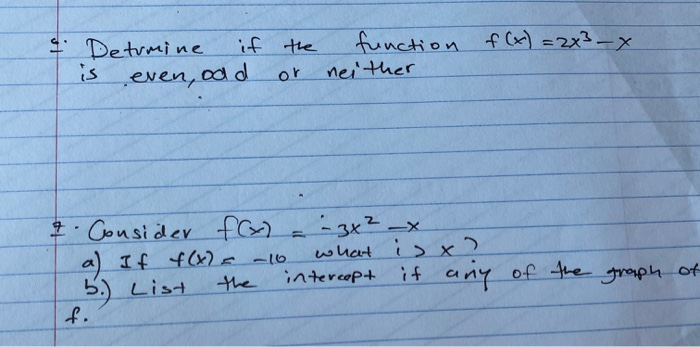& Detumine is if the or function f(x) = 2x² - x neither even, odd 7. Consider f(x) f(x) = - 3x² x a) If f(t) = -10 what is xa List the intercept if any of the graph of f. 5.)

• ### determine whether the given function is even, odd, or neither. f(x)= x^3 - 4

determine whether the given function is even, odd, or neither.f(x)= x^3 - 4x

• ### Determine whether the function x^3+x^2-1 is even, odd or neither

Determine whether the function x^3+x^2-1 is even, odd or neither. I thought it was odd because it has one negative sign is this correct

• ### Determine whether the function of f(x)=[[x+1]] is odd, even, or neither

Determine whether the function of f(x)=[[x+1]] is odd, even, or neither.I figured since it's a step function it automatically couldn't have symmetry and therefore is neither.and doing the whole f(-x) thing:f(-x)=[[-x+1]]which is not equal to f(x) or -f(x). so the answer would be neither, right?

• ### How to determine if function is odd,even or neither

Determine whether the following function is even, odd, or neither. Please show work.f(x)=2-x^2/6x^3

• ### Determine whether the functions are even, odd, or neither

Determine whether the functions are even, odd, or neither.(a) f(x) = x²/√x²+ 1(b) g(x) = x^3 - √ x- 7

• ### Determine whether the function is even, odd or neither

Determine whether the function is even, odd or neitherf(x) = x sin3x

Free Homework App